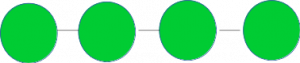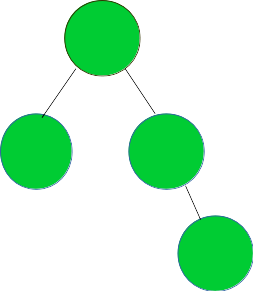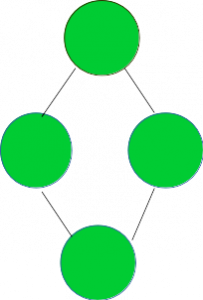# Check if the given graph represents a Bus Topology

Given a graph G, check if it represents a Bus Topology.

A Bus Topology is the one shown in the image below:Examples:

```Input:Output:  YES

Input:Output:  NO
```

## Recommended: Please try your approach on {IDE} first, before moving on to the solution.

A graph of V vertices represents a bus topology if it satisfies the following two conditions:

1. Each node except the stating end ending ones have degree 2 while the starting and ending have degree 1.
2. No of edges = No of Vertices – 1.

The idea is to traverse the graph and check if it satisfies the above two conditions. If yes, then it represents a Bus Topology.

Below is the implementation of the above approach:

 `// CPP program to check if the given graph ` `// represents a bus topology ` `#include ` `using` `namespace` `std; ` ` `  `// A utility function to add an edge in an ` `// undirected graph. ` `void` `addEdge(vector<``int``> adj[], ``int` `u, ``int` `v) ` `{ ` `    ``adj[u].push_back(v); ` `    ``adj[v].push_back(u); ` `} ` ` `  `// A utility function to print the adjacency list ` `// representation of graph ` `void` `printGraph(vector<``int``> adj[], ``int` `V) ` `{ ` `    ``for` `(``int` `v = 0; v < V; ++v) { ` `        ``cout << ``"\n Adjacency list of vertex "` `             ``<< v << ``"\n head "``; ` `        ``for` `(``auto` `x : adj[v]) ` `            ``cout << ``"-> "` `<< x; ` `        ``printf``(``"\n"``); ` `    ``} ` `} ` ` `  `/* Function to return true if the graph represented ` `   ``by the adjacency list represents a bus topology ` `   ``else return false */` `bool` `checkBusTopologyUtil(vector<``int``> adj[], ``int` `V, ``int` `E) ` `{ ` ` `  `    ``// Number of edges should be equal ` `    ``// to (Number of vertices - 1) ` `    ``if` `(E != (V - 1)) ` `        ``return` `false``; ` ` `  `    ``// a single node is termed as a bus topology ` `    ``if` `(V == 1) ` `        ``return` `true``; ` ` `  `    ``int``* vertexDegree = ``new` `int``[V + 1]; ` `    ``memset``(vertexDegree, 0, ``sizeof` `vertexDegree); ` ` `  `    ``// calculate the degree of each vertex ` `    ``for` `(``int` `i = 1; i <= V; i++) { ` `        ``for` `(``auto` `v : adj[i]) { ` `            ``vertexDegree[v]++; ` `        ``} ` `    ``} ` ` `  `    ``// countDegree2 - number of vertices with degree 2 ` `    ``// countDegree1 - number of vertices with degree 1 ` `    ``int` `countDegree2 = 0, countDegree1 = 0; ` `    ``for` `(``int` `i = 1; i <= V; i++) { ` `        ``if` `(vertexDegree[i] == 2) { ` `            ``countDegree2++; ` `        ``} ` `        ``else` `if` `(vertexDegree[i] == 1) { ` `            ``countDegree1++; ` `        ``} ` `        ``else` `{ ` `            ``// if any node has degree other ` `            ``// than 1 or 2, it is ` `            ``// NOT a bus topology ` `            ``return` `false``; ` `        ``} ` `    ``} ` ` `  `    ``// if both necessary conditions as discussed, ` `    ``// satisfy return true ` `    ``if` `(countDegree1 == 2 && countDegree2 == (V - 2)) { ` `        ``return` `true``; ` `    ``} ` `    ``return` `false``; ` `} ` ` `  `// Function to check if the graph represents a bus topology ` `void` `checkBusTopology(vector<``int``> adj[], ``int` `V, ``int` `E) ` `{ ` `    ``bool` `isBus = checkBusTopologyUtil(adj, V, E); ` `    ``if` `(isBus) { ` `        ``cout << ``"YES"` `<< endl; ` `    ``} ` `    ``else` `{ ` `        ``cout << ``"NO"` `<< endl; ` `    ``} ` `} ` ` `  `// Driver code ` `int` `main() ` `{ ` `    ``// Graph 1 ` `    ``int` `V = 5, E = 4; ` `    ``vector<``int``> adj1[V + 1]; ` `    ``addEdge(adj1, 1, 2); ` `    ``addEdge(adj1, 1, 3); ` `    ``addEdge(adj1, 3, 4); ` `    ``addEdge(adj1, 4, 5); ` `    ``checkBusTopology(adj1, V, E); ` ` `  `    ``// Graph 2 ` `    ``V = 4, E = 4; ` `    ``vector<``int``> adj2[V + 1]; ` `    ``addEdge(adj2, 1, 2); ` `    ``addEdge(adj2, 1, 3); ` `    ``addEdge(adj2, 3, 4); ` `    ``addEdge(adj2, 4, 2); ` `    ``checkBusTopology(adj2, V, E); ` ` `  `    ``return` `0; ` `} `

Output:

```YES
NO
```

Time Complexity : O(E), where E is the number of Edges in the graph.

My Personal Notes arrow_drop_upCheck out this Author's contributed articles.

If you like GeeksforGeeks and would like to contribute, you can also write an article using contribute.geeksforgeeks.org or mail your article to contribute@geeksforgeeks.org. See your article appearing on the GeeksforGeeks main page and help other Geeks.

Please Improve this article if you find anything incorrect by clicking on the "Improve Article" button below.